Calculation at the right granularity always needs to be handled carefully when performing data analytics. Especially when data is generated through joining across multiple tables, the denormalization of datasets can add a lot of complications to make accurate calculations challenging. Amazon QuickSight recently launched a new functionality called level-aware calculations (LAC), which enables you to specify any level of granularity at which you want the aggregate functions (at what level to group by) or window functions (in what window to partition by) to be conducted. This brings flexibility and simplification for you to build advanced calculations and powerful analyses. Without LAC, you have to prepare pre-aggregated tables in your original data source, or run queries in the data prep phase to enable those calculations.

In this post, we demonstrate how to create advanced insights using LAC in QuickSight. Before we start walking through the functions, let’s first introduce the important concept of order of evaluation in QuickSight and then talk about LAC in more detail.

## QuickSight order of evaluation

Every time you open or update an analysis, QuickSight evaluates everything that is configured in the analysis in a specific sequence, and translates the configuration into a query that a database engine can run. This is the order of evaluation. The level of complication depends on how many elements are embedded in a visual, multiplied by the number of visuals plus the interactives between the visuals. But if we abstract the concept, the order of evaluation logic can be demonstrated by the following chart.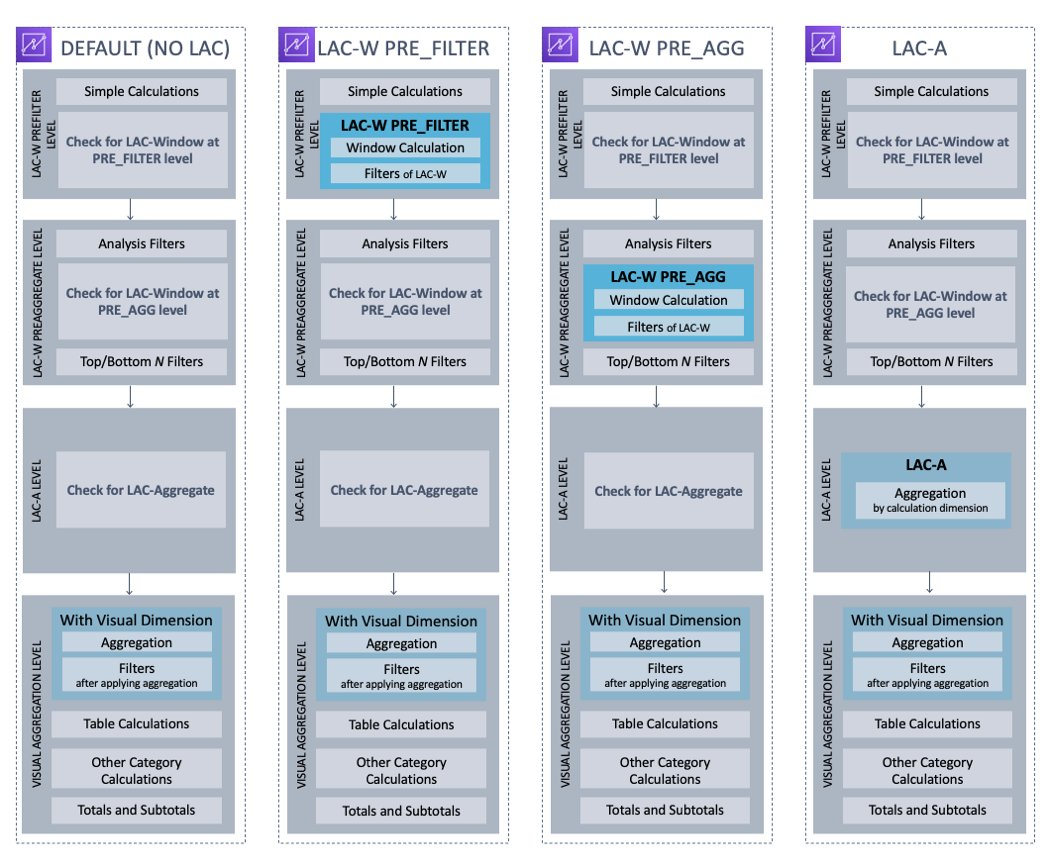In the first DEFAULT route, QuickSight evaluates simple calculations at row level for the raw dataset, then it applies the WHERE filters. After that, based on what dimensions are added to the visual, QuickSight evaluates the aggregation for the selected measures at visual dimensions and applies HAVING filters. Table calculations such as running total or percent of total then get evaluated after the visual is formed, with the subtotal and total calculated last.

Sometimes, you may want the analytical steps to be conducted with a different sequence. For example, you may want to do an aggregation before the data being filtered, or do an aggregation first for some specific dimensions and then aggregate again for the visual dimensions. Based on those different needs, QuickSight offers three variations of order of evaluation (as shown in the preceding chart). Specifically, you can use the key words of PRE_FILTER to add a calculation step before the WHERE filter, use PRE_AGG to add a calculation step before the visual aggregation, or use a whole suite of level-aware calculation-aggregation functions to define an aggregation at independent dimensions, and then aggregate them at the visual dimension (a nested aggregation).

Most of the time, your visuals will include more than one calculated field. You’ll want to be careful to define each of them and understand how they’re interacting with the visuals and with different filters. Applying a filter before or after a window function can generate totally different results and business meanings.

With all the background introduced, now let’s talk about the new LAC functions and their capabilities, and demonstrate several typical use cases.

## Level-aware calculations (LAC)

There are two groups of LAC functions:

• Level-aware calculations-aggregate functions (LAC-A) – These are our newly launched functions. By adding one argument into an existing aggregate function (for example, `sum()`, `max()`, or `count()`), you can define any group-by dimensions you desire for the aggregation. A typical syntax for LAC-A is `sum(measure,[group_field_A])`. With LAC-A, you can add an aggregation step before the visual aggregation. The added layer can be fixed, which is independent of the visual dimension. It also can be dynamically interacting with the visual dimensions. We give some detailed examples later in this post. For a list of supported aggregation functions, refer to Level-aware calculation – aggregate (LAC-A) functions.
• Level-aware calculation-window functions (LAC-W) – These are the existing functions. They used to be called level-aware aggregations (LAA). We recently changed its name to better reflect the function nature, due to underlying difference between window functions and aggregate functions. LAC-W is a group of window functions (such as `sumover()`, `maxover()`, and `denseRank()`) where using a third parameter, you can choose to run the calculation at the PRE_FILTER or PRE-AGG stage. A typical syntax for LAC-W is `sumOver(measure,[partition_field_A],pre_agg)`. For a list of supported window functions, refer to Level-aware calculation – window (LAC-W) functions.

The following high-level diagram shows different branches of LAC. In this post, we mostly focus on the new LAC-A functions.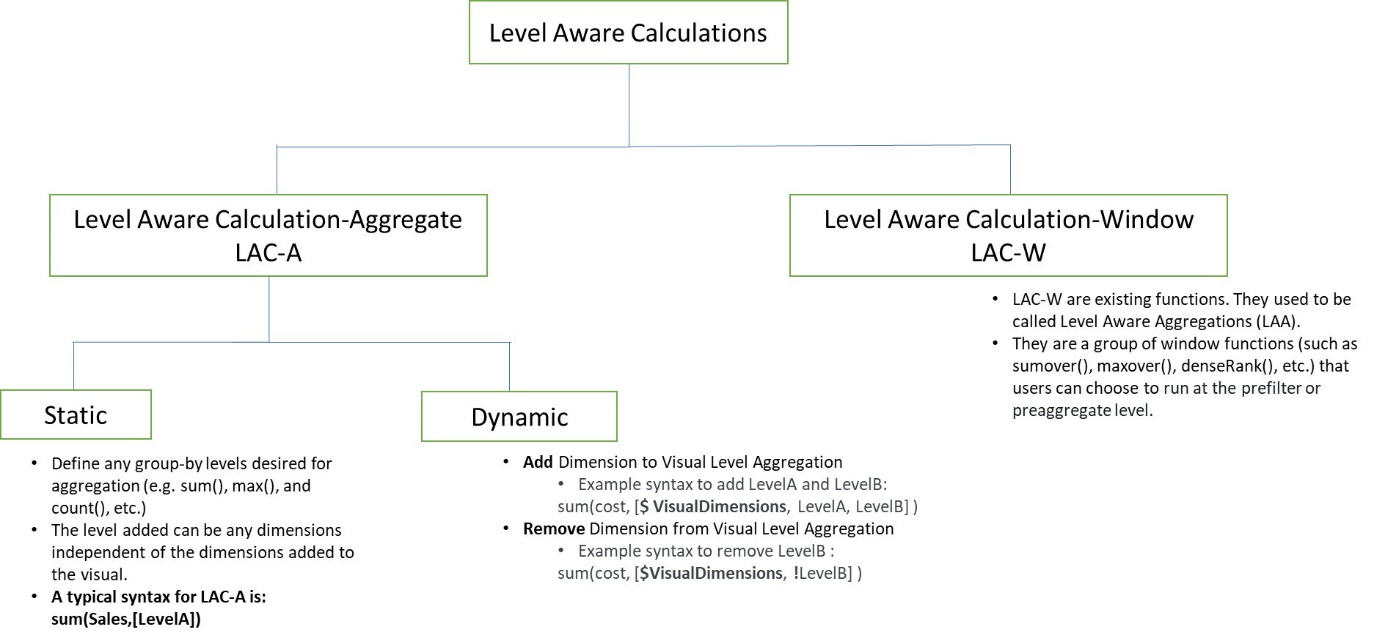With the new LAC-A functions, you can run two layers of aggregation calculations. This offers the following benefits:

• Run aggregation calculations that are independent of the group-by fields in the visual calculation
• Run aggregation calculations for the dimensions that are NOT in the visual
• Remove duplication of raw data before running calculations
• Run aggregation calculations with nested group-by fields dynamically adapting to visual group-by fields

Let’s explore how we can achieve those benefits by demonstrating a few use cases.

## Use case #1: Identify orders where actual ordered quantity for a product is higher than the average quantity

In this case, our visual is at the order level; however, we want to compute the average quantity of a product and use that to display the difference at each individual row/order level. With the LAC-A function, we can easily create an aggregation that is independent of the level in the visual.

We first compute the average quantity sold at product level using the expression of `avg(Quantity,[Product])`. To do so, change the visual-level aggregation to Average. In this case, visual-level aggregation doesn’t matter because we have `product` as a column and the LAC-A are at the same level. In the result table, the average quantity value for `product` is repeated across all orders because this is computed at the product level.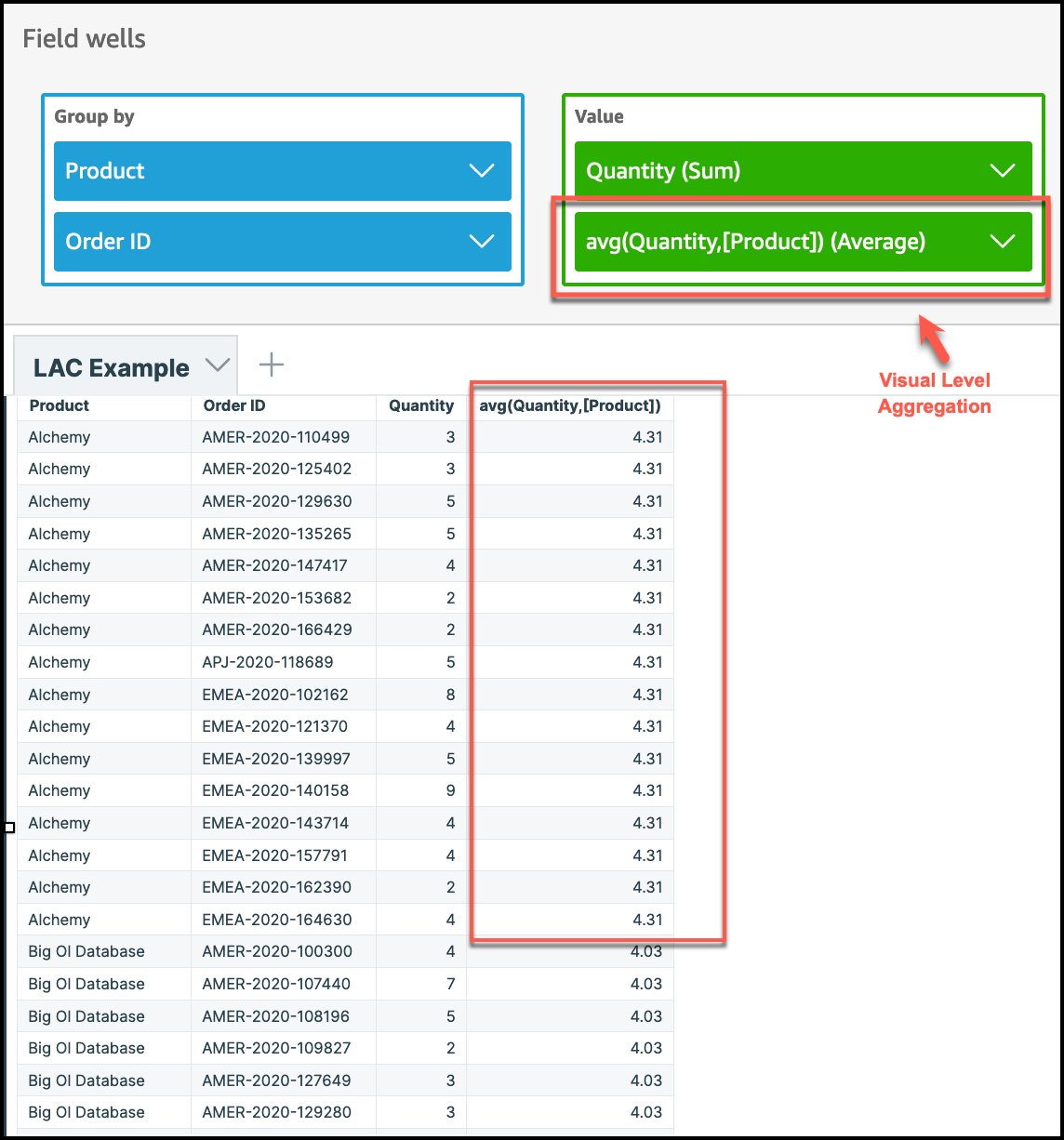Now that we have computed the average quantity at the product level, we can extend this to compute the difference between actual quantity ordered and the average quantity of product using the expression `sum(Quantity) - avg(avg(Quantity,[Product]))`. This computed difference can then be used to conditionally format the view to highlight orders that have quantity higher than the average quantity of a product.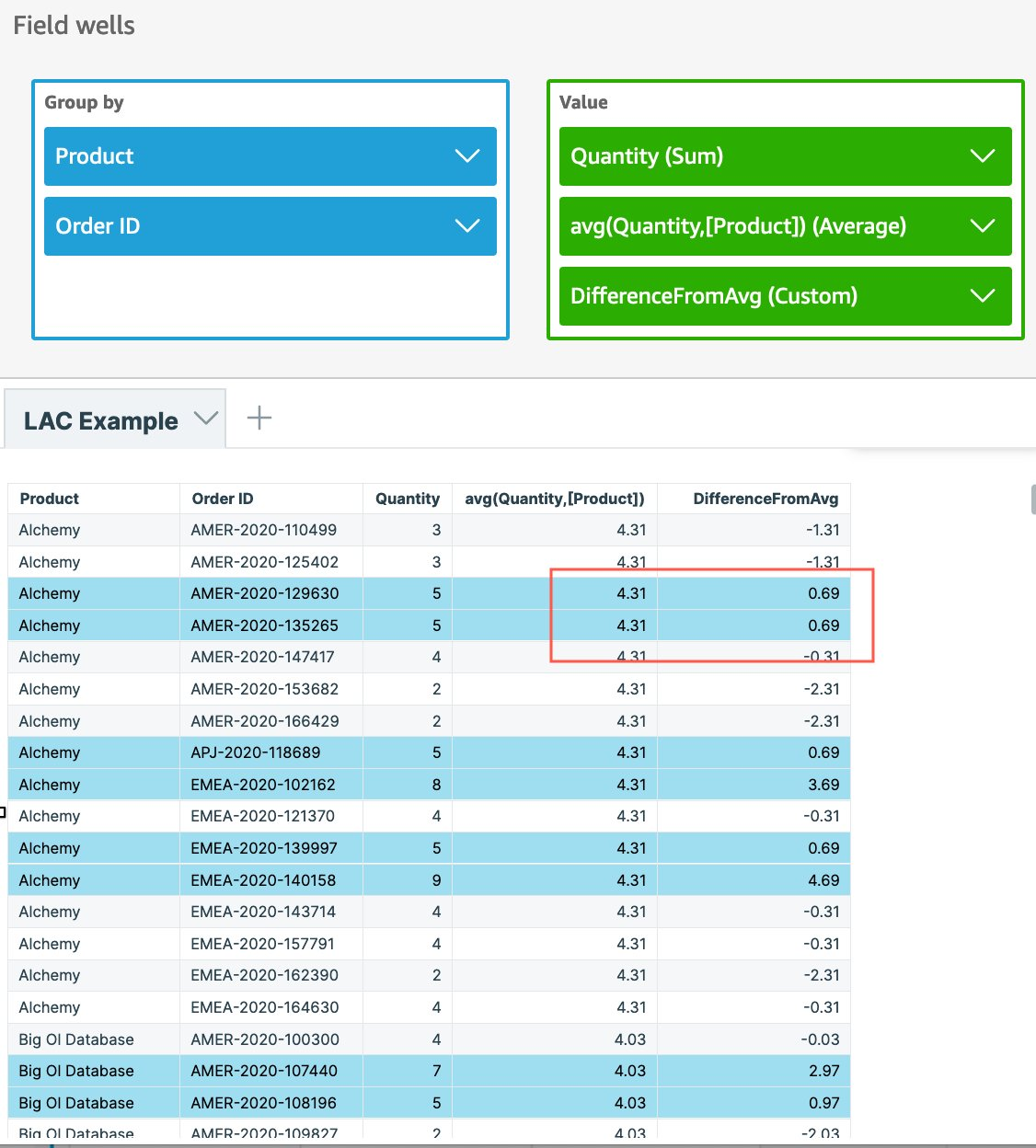As seen in this example, although the visual was at the order level, we easily created an aggregation like average quantity of product, which is independent of the level in the visual, and used that to display the difference at each individual row/order level.

## Use case #2: Identify the average of total country sales by region

Here we want to aggregate the sales for each country and then compute the average of country-level sales at region level using the same dataset.

With the LAC-A function, we can easily create an aggregation at a dimension level that is NOT in the visual. In this example, although `country` is not included in the visual, the LAC-A function first aggregates the sales at the country level and then the visual-level calculation generates the average number for each region. Within QuickSight, we can implement this in two ways.

### Option 1: Nest the LAC-A with visual-level aggregate functions

Create a calculated column to compute sales at country level by using the expression of `sum(Sales,[Country] )`, then add the calculation to the visual and change the aggregation to Average, as shown in the following screenshot. Note that if we don’t use LAC-A to specify the level, the average sales are calculated at the lowest granular level (the base level of the dataset) for each region. That’s why the numbers are significantly smaller for the `sales` column.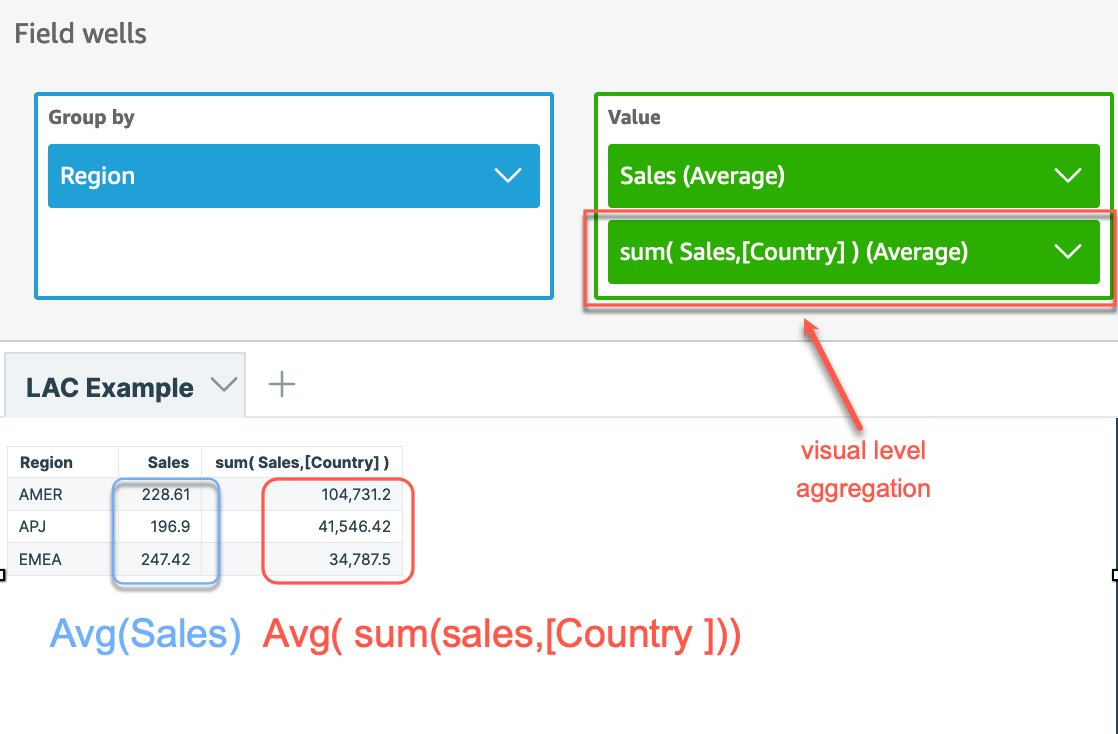### Option 2: Use LAC-A combined with other aggregate functions and nest them in the calculated column

Create a calculated column to compute sales at country level by using the expression `sum(Sales,[Country])` and then nest that with additional aggregation, in this case Average, by using the expression `avg(sum(Sales,[Country]))`.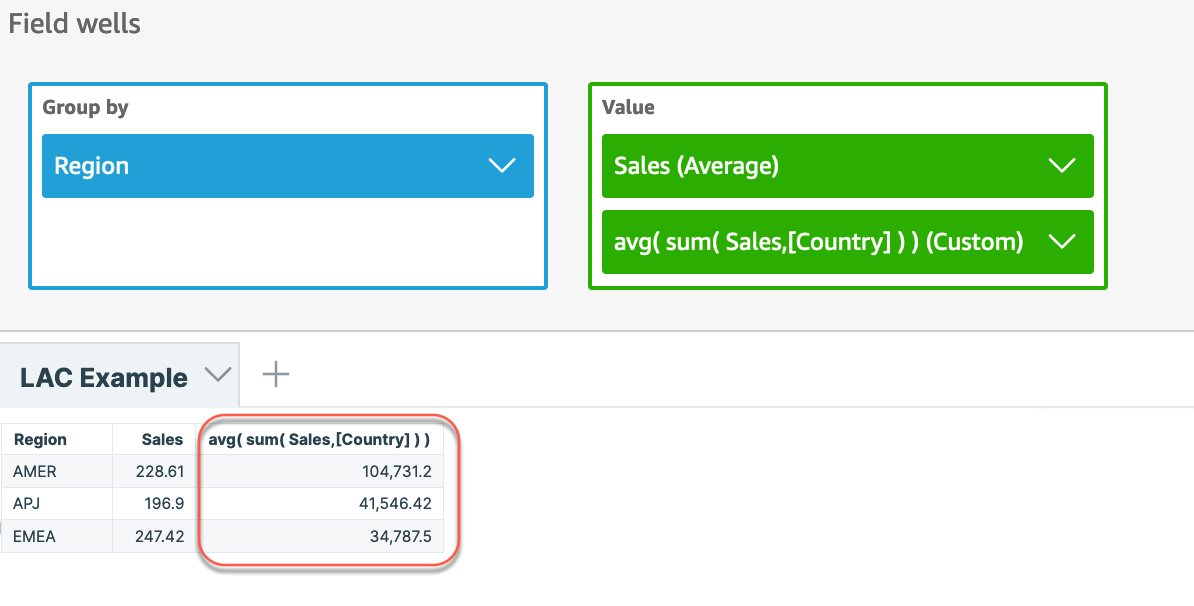## Use case #3: Calculate total and average for a denormalized dataset with duplications

LAC-A calculations are designed to effectively handle duplicates in data while performing computation. It allows you to perform computations like average without the need for explicit handling of duplicates in data.

Consider a dataset that has employee and project details along with each employee’s salary. An employee can be associated with multiple projects, as shown in the following example.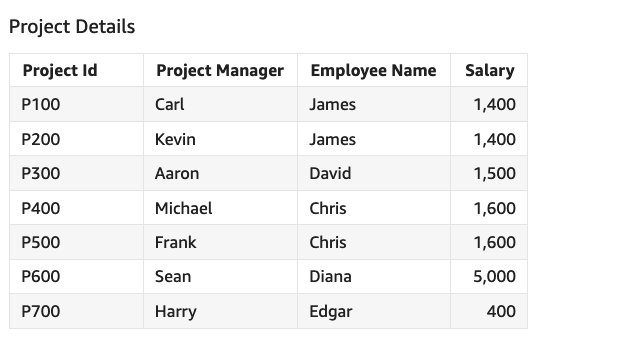Now let’s calculate total employee salary, average employee salary, and minimum and maximum salary using this sample dataset.

To compute total and average, we have to consider each employee’s salary just once even though an employee can be part of multiple projects and the salary for the employee can get duplicated across these projects. We can easily achieve this using LAC-A to compute the maximum of the salary at the employee level and then use that to compute the total and average.

Create a calculated column called Total Salary using the expression `sum(max(Salary,[{Employee Name}]))` and create another calculated column called Average Salary using `avg(max(Salary,[{Employee Name}]))`. We can easily calculate Min Salary using the expression `min(Salary)` and Max Salary using `max(Salary)`.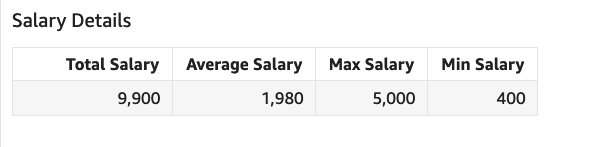If we try to solve this without using LAC-A, we have to explicitly handle salary duplication in our calculation, and we have to go through multiple steps to get to the final result. Refer to the QuickSight Community blog for a similar use case.

## Dynamic group keys for LAC-A

In addition to defining a static level of aggregation as seen in the preceding examples, you can also dynamically add or remove dimensions from the visual level group-by fields. The following are example syntax for a dynamic level:

• Add dimensions to the visual dimensions with `sum(cost, [\$VisualDimensions, LevelA, LevelB])`
• Remove dimensions from the visual dimensions with `sum(cost, [\$VisualDimensions, !LevelC, !LevelD])`

This capability brings a lot of flexibility and scalability for you to make the LAC even more powerful. Firstly, you can define one LAC calculated field and reuse it across multiple visuals with similar business intents. Additionally, if you’re building the visuals and keep adding or deleting the visual fields, you don’t need to edit the LAC-A calculated field each time, and the LAC-A will automatically adjust to the visual dimensions and give the right output.

## Use case #4: Identify average sales of customers within each region or country

To compute this, we can use the dynamic expression `Sum(Sales, [\$VisualDimensions, Customers])`. This is because each customer has purchases in more than one region. We need to calculate the customer average sales only within each region. We can reuse the same expression in a different visual with `country` as the visual dimension if we want to calculate average sales of customers within each country.

Use Average as the visual-level metric with Group by as Region to get the region-level average. In this case, `“\$visualDimensions”` is adapted to `Region`. So the expression is equivalent to `Sum(Sales, [Region, Customers])` in this visual.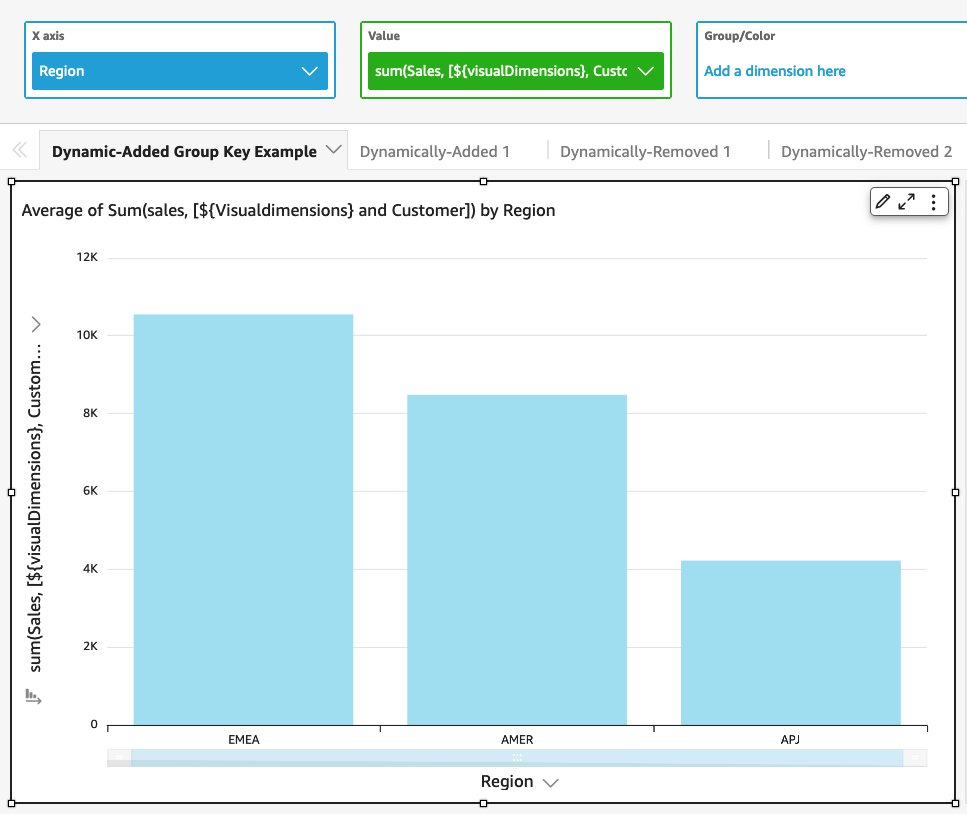If you have a similar visual with the `Country` dimension, then the dynamic expression is equivalent to `Sum(Sales, [Country, Customers])`. Reusing the same expression in different visuals saves us a lot of time, especially when we want to build similar visuals with slightly different business context.

## Use case #5: Identify the sales percentage of each subregion compared to region level

In this example, we want to calculate the percentage of sales for each subregion, comparing with the total region sales. You can use the fixed dimension as we mentioned before, but imagine a situation when you want to include more dimensions into the visual such as product, year, or supplier. Using the dynamic group key by removing only {subregion} from the visual dimensions makes the exploration process much easier and quicker.

First, create an expression to calculate the sum of sales without the subregion level using `sum(Sales, [\$visualDimensions, !subregion])`, then calculate the percentage using `sum(Sales) / sum(sum(Sales, [\$visualDimensions, !subregion]))`.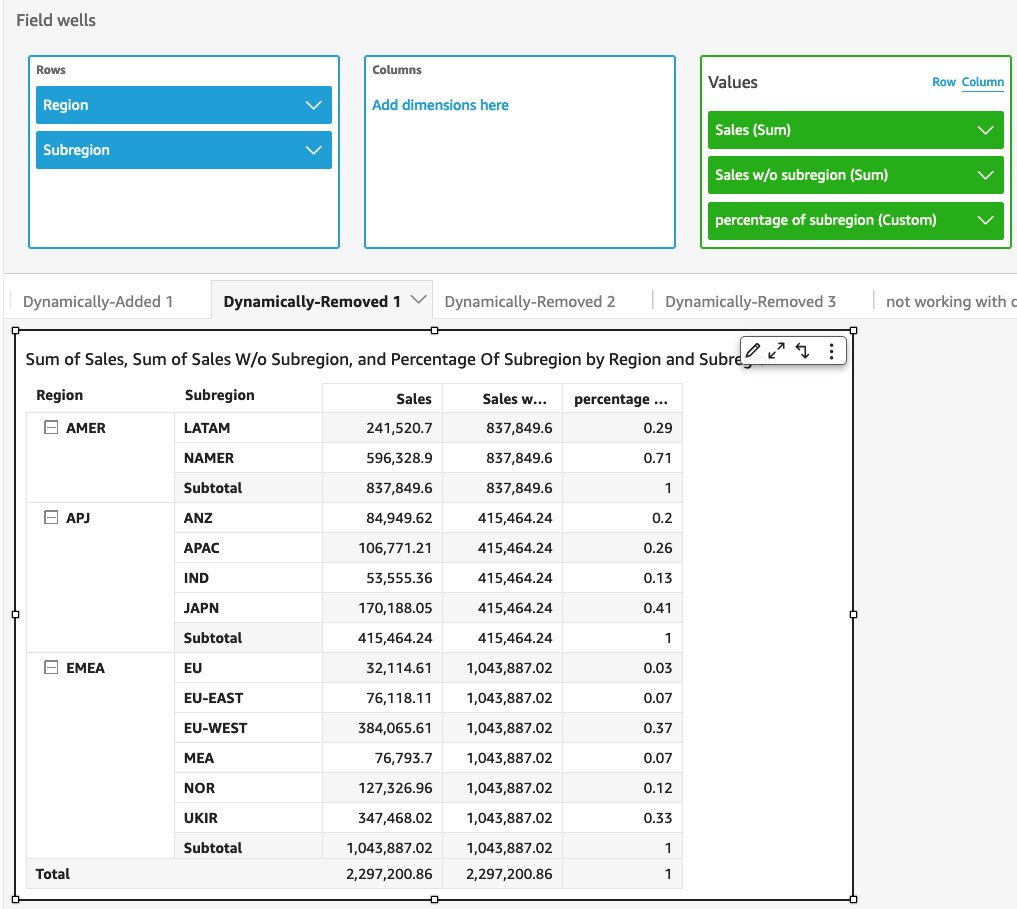## Conclusion

In this post, we introduced new QuickSight LAC-A functions, which enable powerful and advanced aggregations with user-defined dimensions. We introduced the QuickSight order of evaluation, and walked through three use cases for LAC-A static keys and two use cases of LAC-A dynamic keys. Level-aware calculations are now generally available in all supported QuickSight Regions.

We look forward to your feedback and stories on how you apply these calculations for your business needs.Karthik Tharmarajan is a Senior Specialist Solutions Architect for Amazon QuickSight. Karthik has over 15 years of experience implementing enterprise business intelligence (BI) solutions and specializes in integration of BI solutions with business applications and enable data-driven decisions.Emily Zhu is a Senior Product Manager-Tech at Amazon QuickSight, AWS’s cloud-native, fully managed SaaS BI service. She leads the development of the QuickSight analytics and query experience. Before joining AWS, she worked in the Amazon Prime Air drone delivery program and the Boeing company as senior strategist for several years. Emily is passionate about the potential of cloud-based BI solutions and looks forward to helping customers advance in their data-driven strategy making.Feng Han is a Software Development Manager in AWS QuickSight Query Platform team. He focuses on query generation platform and advanced function calculation, and is leading the team to next generation of calculation engine.